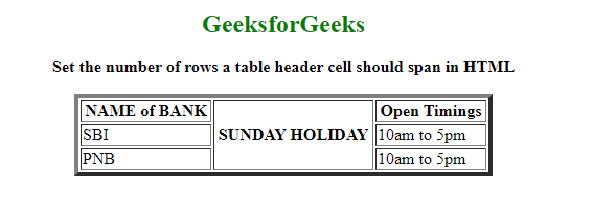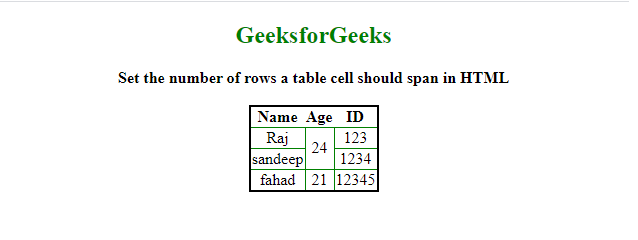Related Articles

# How to set the number of rows a table cell should span in HTML ?

• Last Updated : 26 May, 2021

In this article, we will show how multiple rows are spanned by a table header cell. The task can be done by using the rowspan attribute while using the <th> tag. This property is used to merge one or more cells as well as increase the height of the single header cell automatically.

Syntax:

`<th rowspan = "value">table content...</th>`

Example:

## HTML

 ```<``html``>``  ``<``body``>``    ``<``center``>``      ``<``h2` `style``=``"color: green"``>GeeksforGeeks`` ` `      ``<``b``> ``       ``Set the number of rows a table header ``       ``cell should span in HTML ``      ````      ``<``table` `border``=``"4"` `width``=``"400"``>``        ``<``tr``>``          ``<``th``>NAME of BANK``          ``<``th` `rowspan``=``"3"``>SUNDAY HOLIDAY``          ``<``th``>Open Timings``        ```` ` `        ``<``tr``>``          ``<``td``>SBI``          ``<``td``>10am to 5pm``        ```` ` `        ``<``tr``>``          ``<``td``>PNB``          ``<``td``>10am to 5pm``        ````      ````    ````  `````

Output:Example 2: In this example. we will learn, how rows can be made to span over multiple cells.

The task can be done by using the rowspan attribute when using the <td> tag within <tr> tag.  It allows the single table cell to span the height of more than one cell. It contains a numeric value that defines the number of rows that should be spanned.

Syntax:

```<tr>
<td rowspan = "value">table column content...</td>
</tr>```

## HTML

 ```<``html``>``  ``<``head``>``    ``<``style``>``      ``table {``        ``border: 2px solid black;``        ``border-collapse: collapse;``        ``padding: 6px;``      ``}``      ``td {``        ``border: 1px solid green;``        ``text-align: center;``      ``}``    ````  ```` ` `  ``<``body` `style``=``"text-align: center"``>``    ``<``center``>``      ``<``h2` `style``=``"color: green"``>GeeksforGeeks``      ``<``b``> ``       ``Set the number of rows a table``       ``cell should span in HTML ``      ```` ` `      ``<``br` `/>``      ``<``table``>``        ``<``tr``>``          ``<``th``>Name``          ``<``th``>Age``          ``<``th``>ID``        ````        ``<``tr``>``          ``<``td``>Raj``          ````          ``<``td` `rowspan``=``"2"``>24``          ``<``td``>123``        ````        ``<``tr``>``          ``<``td``>sandeep``          ``<``td``>1234``        ```` ` `        ``<``tr``>``          ``<``td``>fahad``          ``<``td``>21``          ``<``td``>12345``        ````      ````    ````  ``````              `

Output:Attention reader! Don’t stop learning now. Get hold of all the important Comcompetitivepetitve Programming concepts with the Web Design for Beginners | HTML  course.

My Personal Notes arrow_drop_up